Publicité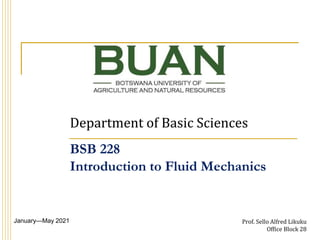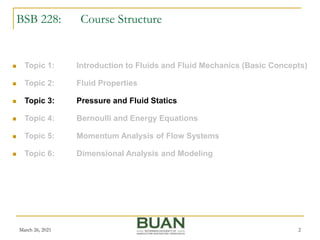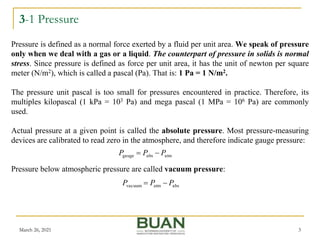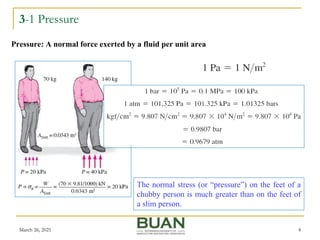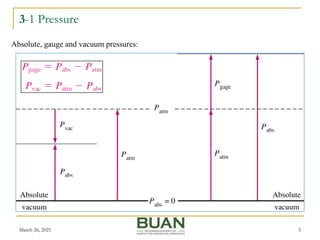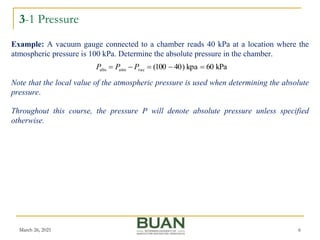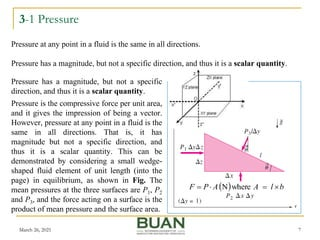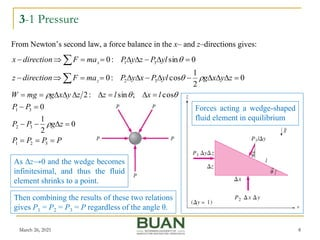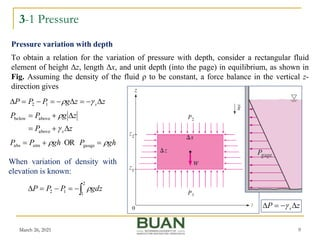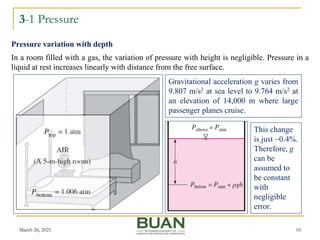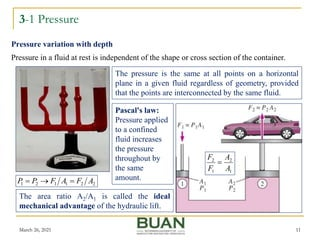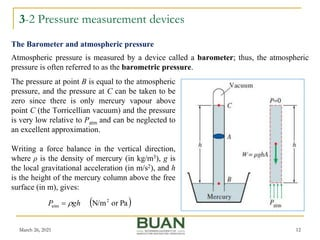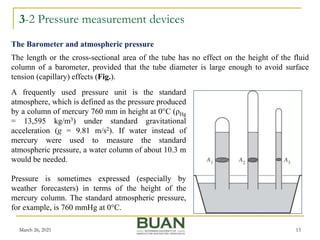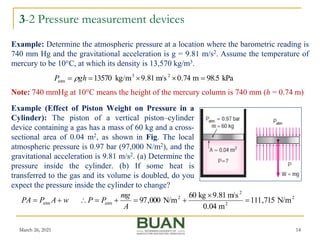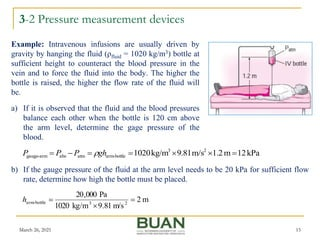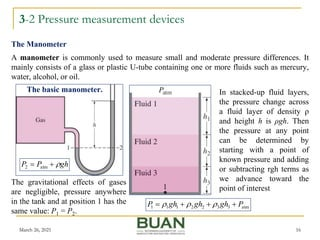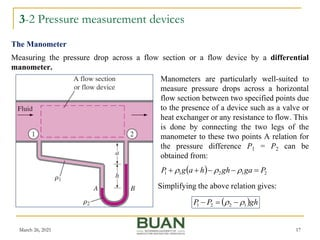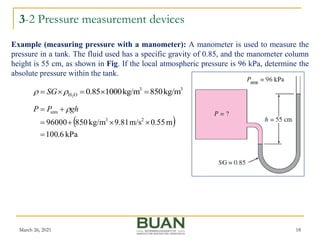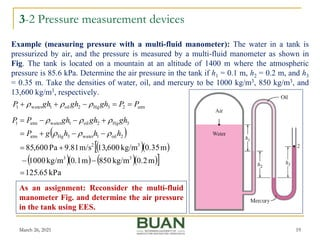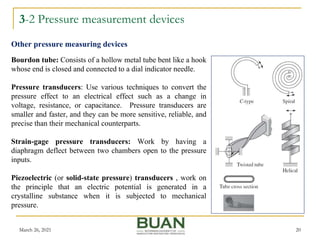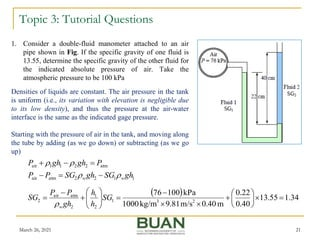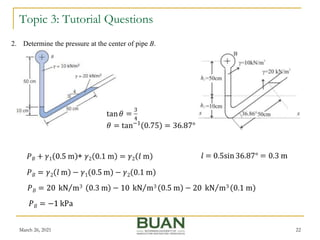1 sur 22
Publicité

### 03 1 bsb 228 pressure and pressure measurement

1. Department of Basic Sciences January—May 2021 Prof. Sello Alfred Likuku Office Block 28 BSB 228 Introduction to Fluid Mechanics
2. BSB 228: Course Structure March 26, 2021 2  Topic 1: Introduction to Fluids and Fluid Mechanics (Basic Concepts)  Topic 2: Fluid Properties  Topic 3: Pressure and Fluid Statics  Topic 4: Bernoulli and Energy Equations  Topic 5: Momentum Analysis of Flow Systems  Topic 6: Dimensional Analysis and Modeling
3. 3-1 Pressure March 26, 2021 3 Pressure is defined as a normal force exerted by a fluid per unit area. We speak of pressure only when we deal with a gas or a liquid. The counterpart of pressure in solids is normal stress. Since pressure is defined as force per unit area, it has the unit of newton per square meter (N/m2), which is called a pascal (Pa). That is: 1 Pa = 1 N/m2. The pressure unit pascal is too small for pressures encountered in practice. Therefore, its multiples kilopascal (1 kPa = 103 Pa) and mega pascal (1 MPa = 106 Pa) are commonly used. Actual pressure at a given point is called the absolute pressure. Most pressure-measuring devices are calibrated to read zero in the atmosphere, and therefore indicate gauge pressure: Pressure below atmospheric pressure are called vacuum pressure: atm abs gauge P P P   abs atm vacuum P P P  
4. 3-1 Pressure March 26, 2021 4 Pressure: A normal force exerted by a fluid per unit area The normal stress (or “pressure”) on the feet of a chubby person is much greater than on the feet of a slim person.
5. 3-1 Pressure March 26, 2021 5 Absolute, gauge and vacuum pressures:
6. 3-1 Pressure March 26, 2021 6 Example: A vacuum gauge connected to a chamber reads 40 kPa at a location where the atmospheric pressure is 100 kPa. Determine the absolute pressure in the chamber. Note that the local value of the atmospheric pressure is used when determining the absolute pressure. kPa 60 kpa ) 40 100 ( vac atm abs      P P P Throughout this course, the pressure P will denote absolute pressure unless specified otherwise.
7. 3-1 Pressure March 26, 2021 7 Pressure at any point in a fluid is the same in all directions. Pressure has a magnitude, but not a specific direction, and thus it is a scalar quantity. Pressure has a magnitude, but not a specific direction, and thus it is a scalar quantity. Pressure is the compressive force per unit area, and it gives the impression of being a vector. However, pressure at any point in a fluid is the same in all directions. That is, it has magnitude but not a specific direction, and thus it is a scalar quantity. This can be demonstrated by considering a small wedge- shaped fluid element of unit length (into the page) in equilibrium, as shown in Fig. The mean pressures at the three surfaces are P1, P2 and P3, and the force acting on a surface is the product of mean pressure and the surface area.   b l A A P F     where N
8. P P P P z g P P P P l x l z z y x g mg W                   3 2 1 3 2 3 1 0 2 1 0 cos ; sin : 2     3-1 Pressure March 26, 2021 8 As Δz→0 and the wedge becomes infinitesimal, and thus the fluid element shrinks to a point. From Newton’s second law, a force balance in the x– and z–directions gives: 0 2 1 cos : 0 0 sin : 0 3 2 3 1                         z y x g yl P x y P ma F direction z yl P z y P ma F direction x z x    Forces acting a wedge-shaped fluid element in equilibrium Then combining the results of these two relations gives P1 = P2 = P3 = P regardless of the angle θ.
9. 3-1 Pressure March 26, 2021 9 To obtain a relation for the variation of pressure with depth, consider a rectangular fluid element of height Δz, length Δx, and unit depth (into the page) in equilibrium, as shown in Fig. Assuming the density of the fluid ρ to be constant, a force balance in the vertical z- direction gives Pressure variation with depth z z g P P P s           1 2 z P s     z P z g P P s        above above below gh P gh P P      gauge atm abs OR When variation of density with elevation is known:       2 1 1 2 gdz P P P 
10. 3-1 Pressure March 26, 2021 10 In a room filled with a gas, the variation of pressure with height is negligible. Pressure in a liquid at rest increases linearly with distance from the free surface. Pressure variation with depth Gravitational acceleration g varies from 9.807 m/s2 at sea level to 9.764 m/s2 at an elevation of 14,000 m where large passenger planes cruise. This change is just ~0.4%. Therefore, g can be assumed to be constant with negligible error.
11. 3-1 Pressure March 26, 2021 11 Pressure in a fluid at rest is independent of the shape or cross section of the container. Pressure variation with depth The pressure is the same at all points on a horizontal plane in a given fluid regardless of geometry, provided that the points are interconnected by the same fluid. Pascal's law: Pressure applied to a confined fluid increases the pressure throughout by the same amount. 2 2 1 1 2 1 A F A F P P    The area ratio A2/A1 is called the ideal mechanical advantage of the hydraulic lift. 1 2 1 2 A A F F 
12. 3-2 Pressure measurement devices March 26, 2021 12 Atmospheric pressure is measured by a device called a barometer; thus, the atmospheric pressure is often referred to as the barometric pressure. The Barometer and atmospheric pressure The pressure at point B is equal to the atmospheric pressure, and the pressure at C can be taken to be zero since there is only mercury vapour above point C (the Torricellian vacuum) and the pressure is very low relative to Patm and can be neglected to an excellent approximation. Writing a force balance in the vertical direction, where ρ is the density of mercury (in kg/m3), g is the local gravitational acceleration (in m/s2), and h is the height of the mercury column above the free surface (in m), gives:   Pa or N/m2 atm gh P  
13. 3-2 Pressure measurement devices March 26, 2021 13 The length or the cross-sectional area of the tube has no effect on the height of the fluid column of a barometer, provided that the tube diameter is large enough to avoid surface tension (capillary) effects (Fig.). The Barometer and atmospheric pressure A frequently used pressure unit is the standard atmosphere, which is defined as the pressure produced by a column of mercury 760 mm in height at 0°C (ρHg = 13,595 kg/m3) under standard gravitational acceleration (g = 9.81 m/s2). If water instead of mercury were used to measure the standard atmospheric pressure, a water column of about 10.3 m would be needed. Pressure is sometimes expressed (especially by weather forecasters) in terms of the height of the mercury column. The standard atmospheric pressure, for example, is 760 mmHg at 0°C.
14. 3-2 Pressure measurement devices March 26, 2021 14 Note: 740 mmHg at 10°C means the height of the mercury column is 740 mm (h = 0.74 m) Example: Determine the atmospheric pressure at a location where the barometric reading is 740 mm Hg and the gravitational acceleration is g = 9.81 m/s2. Assume the temperature of mercury to be 10°C, at which its density is 13,570 kg/m3. kPa 98.5 m 74 . 0 m/s 81 . 9 kg/m 13570 2 3 atm      gh P  Example (Effect of Piston Weight on Pressure in a Cylinder): The piston of a vertical piston–cylinder device containing a gas has a mass of 60 kg and a cross- sectional area of 0.04 m2, as shown in Fig. The local atmospheric pressure is 0.97 bar (97,000 N/m2), and the gravitational acceleration is 9.81 m/s2. (a) Determine the pressure inside the cylinder. (b) If some heat is transferred to the gas and its volume is doubled, do you expect the pressure inside the cylinder to change? w A P PA   atm 2 2 2 2 atm N/m 715 , 111 m 04 . 0 m/s 81 . 9 kg 60 N/m 000 , 97        A mg P P
15. 3-2 Pressure measurement devices March 26, 2021 15 Example: Intravenous infusions are usually driven by gravity by hanging the fluid (ρfluid = 1020 kg/m3) bottle at sufficient height to counteract the blood pressure in the vein and to force the fluid into the body. The higher the bottle is raised, the higher the flow rate of the fluid will be. kPa 12 m 2 . 1 m/s 81 . 9 kg/m 1020 2 3 bottle - arm atm abs arm - gauge        gh P P P  a) If it is observed that the fluid and the blood pressures balance each other when the bottle is 120 cm above the arm level, determine the gage pressure of the blood. b) If the gauge pressure of the fluid at the arm level needs to be 20 kPa for sufficient flow rate, determine how high the bottle must be placed. m 2 m/s 81 . 9 kg/m 1020 Pa 000 , 20 2 3 bottle - arm    h
16. 3-2 Pressure measurement devices March 26, 2021 16 A manometer is commonly used to measure small and moderate pressure differences. It mainly consists of a glass or plastic U-tube containing one or more fluids such as mercury, water, alcohol, or oil. The Manometer In stacked-up fluid layers, the pressure change across a fluid layer of density ρ and height h is ρgh. Then the pressure at any point can be determined by starting with a point of known pressure and adding or subtracting rgh terms as we advance toward the point of interest The basic manometer. gh P P    atm 2 atm 3 3 2 2 1 1 1 P gh gh gh P        The gravitational effects of gases are negligible, pressure anywhere in the tank and at position 1 has the same value: P1 = P2.
17. 3-2 Pressure measurement devices March 26, 2021 17 Measuring the pressure drop across a flow section or a flow device by a differential manometer. The Manometer Manometers are particularly well-suited to measure pressure drops across a horizontal flow section between two specified points due to the presence of a device such as a valve or heat exchanger or any resistance to flow. This is done by connecting the two legs of the manometer to these two points A relation for the pressure difference P1 = P2 can be obtained from:   2 1 2 1 1 P ga gh h a g P          gh P P 1 2 2 1      Simplifying the above relation gives:
18. 3-2 Pressure measurement devices March 26, 2021 18 Example (measuring pressure with a manometer): A manometer is used to measure the pressure in a tank. The fluid used has a specific gravity of 0.85, and the manometer column height is 55 cm, as shown in Fig. If the local atmospheric pressure is 96 kPa, determine the absolute pressure within the tank.   kPa 6 . 100 m 55 . 0 m/s 81 . 9 kg/m 850 96000 2 3 atm        gh P P  3 3 O H kg/m 850 kg/m 1000 85 . 0 2        SG
19. 3-2 Pressure measurement devices March 26, 2021 19 Example (measuring pressure with a multi-fluid manometer): The water in a tank is pressurized by air, and the pressure is measured by a multi-fluid manometer as shown in Fig. The tank is located on a mountain at an altitude of 1400 m where the atmospheric pressure is 85.6 kPa. Determine the air pressure in the tank if h1 = 0.1 m, h2 = 0.2 m, and h3 = 0.35 m. Take the densities of water, oil, and mercury to be 1000 kg/m3, 850 kg/m3, and 13,600 kg/m3, respectively. atm 2 3 Hg 2 oil 1 water 1 P P gh gh gh P                     kPa 65 . 125 m .2 0 kg/m 50 8 m .1 0 kg/m 1000 m .35 0 kg/m 600 , 13 m/s 81 . 9 Pa 600 , 85 3 3 3 2 2 oil 1 water 3 Hg atm 3 Hg 2 oil 1 water atm 1              h h h g P gh gh gh P P       As an assignment: Reconsider the multi-fluid manometer Fig. and determine the air pressure in the tank using EES.
20. 3-2 Pressure measurement devices March 26, 2021 20 Bourdon tube: Consists of a hollow metal tube bent like a hook whose end is closed and connected to a dial indicator needle. Pressure transducers: Use various techniques to convert the pressure effect to an electrical effect such as a change in voltage, resistance, or capacitance. Pressure transducers are smaller and faster, and they can be more sensitive, reliable, and precise than their mechanical counterparts. Strain-gage pressure transducers: Work by having a diaphragm deflect between two chambers open to the pressure inputs. Piezoelectric (or solid-state pressure) transducers , work on the principle that an electric potential is generated in a crystalline substance when it is subjected to mechanical pressure. Other pressure measuring devices
21. Topic 3: Tutorial Questions March 26, 2021 21 1. Consider a double-fluid manometer attached to an air pipe shown in Fig. If the specific gravity of one fluid is 13.55, determine the specific gravity of the other fluid for the indicated absolute pressure of air. Take the atmospheric pressure to be 100 kPa 1 1 2 2 atm air atm 2 2 1 1 air gh SG gh SG P P P gh gh P w w           Densities of liquids are constant. The air pressure in the tank is uniform (i.e., its variation with elevation is negligible due to its low density), and thus the pressure at the air-water interface is the same as the indicated gage pressure.   34 . 1 55 . 13 40 . 0 22 . 0 m 40 . 0 m/s 81 . 9 kg/m 1000 kPa 100 76 2 3 1 2 1 2 atm air 2                         SG h h gh P P SG w  Starting with the pressure of air in the tank, and moving along the tube by adding (as we go down) or subtracting (as we go up)
22. Topic 3: Tutorial Questions March 26, 2021 22 2. Determine the pressure at the center of pipe B.
Publicité